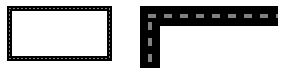# Rectangle Struct

## Definition

Stores a set of four integers that represent the location and size of a rectangle.

``public value class Rectangle : IEquatable<System::Drawing::Rectangle>``
``public value class Rectangle``
``````[System.ComponentModel.TypeConverter("System.Drawing.RectangleConverter, System.Drawing, Version=4.0.0.0, Culture=neutral, PublicKeyToken=b03f5f7f11d50a3a")]
public struct Rectangle : IEquatable<System.Drawing.Rectangle>``````
``public struct Rectangle``
``public struct Rectangle : IEquatable<System.Drawing.Rectangle>``
``````[System.ComponentModel.TypeConverter(typeof(System.Drawing.RectangleConverter))]
[System.Runtime.InteropServices.ComVisible(true)]
[System.Serializable]
public struct Rectangle``````
``````[<System.ComponentModel.TypeConverter("System.Drawing.RectangleConverter, System.Drawing, Version=4.0.0.0, Culture=neutral, PublicKeyToken=b03f5f7f11d50a3a")>]
type Rectangle = struct``````
``type Rectangle = struct``
``````[<System.ComponentModel.TypeConverter(typeof(System.Drawing.RectangleConverter))>]
[<System.Runtime.InteropServices.ComVisible(true)>]
[<System.Serializable>]
type Rectangle = struct``````
``````Public Structure Rectangle
Implements IEquatable(Of Rectangle)``````
``Public Structure Rectangle``
Inheritance
Rectangle
Attributes
Implements

## Examples

The following example draws a rectangle with its upper-left corner at (10, 10). The rectangle has a width of 100 and a height of 50. The second argument passed to the Pen constructor indicates that the pen width is 5 pixels.

When the rectangle is drawn, the pen is centered on the rectangle's boundary. Because the pen width is 5, the sides of the rectangle are drawn 5 pixels wide, such that 1 pixel is drawn on the boundary itself, 2 pixels are drawn on the inside, and 2 pixels are drawn on the outside. For more details on pen alignment, see How to: Set Pen Width and Alignment.

The following illustration shows the resulting rectangle. The dotted lines show where the rectangle would have been drawn if the pen width had been one pixel. The enlarged view of the upper-left corner of the rectangle shows that the thick black lines are centered on those dotted lines.The example is designed for use with Windows Forms, and it requires PaintEventArgs `e`, which is a parameter of the Paint event handler.

``````Pen blackPen = new Pen(Color.FromArgb(255, 0, 0, 0), 5);
e.Graphics.DrawRectangle(blackPen, 10, 10, 100, 50);
``````
``````Dim blackPen As New Pen(Color.FromArgb(255, 0, 0, 0), 5)
e.Graphics.DrawRectangle(blackPen, 10, 10, 100, 50)
``````

## Remarks

A rectangle is defined by its Width, Height, and upper-left corner represented by the Location property.

To draw rectangles, you need a Graphics object and a Pen object. The Graphics object provides the DrawRectangle method, and the Pen object stores features of the line, such as color and width. The units the rectangle is drawn in is determined by the PageUnit and PageScale properties of the graphics object used for drawing. The default unit is pixels.

To draw a Rectangle filled with color, you need a Graphics object and an object derived from Brush such as SolidBrush or LinearGradientBrush. The Graphics object provides the FillRectangle method and the Brush object provides the color and fill information.

For more advanced shapes, use a Region object.

## Constructors

 Initializes a new instance of the Rectangle class with the specified location and size. Initializes a new instance of the Rectangle class with the specified location and size.

## Fields

 Represents a Rectangle structure with its properties left uninitialized.

## Properties

 Gets the y-coordinate that is the sum of the Y and Height property values of this Rectangle structure. Gets or sets the height of this Rectangle structure. Tests whether all numeric properties of this Rectangle have values of zero. Gets the x-coordinate of the left edge of this Rectangle structure. Gets or sets the coordinates of the upper-left corner of this Rectangle structure. Gets the x-coordinate that is the sum of X and Width property values of this Rectangle structure. Gets or sets the size of this Rectangle. Gets the y-coordinate of the top edge of this Rectangle structure. Gets or sets the width of this Rectangle structure. Gets or sets the x-coordinate of the upper-left corner of this Rectangle structure. Gets or sets the y-coordinate of the upper-left corner of this Rectangle structure.

## Methods

 Converts the specified RectangleF structure to a Rectangle structure by rounding the RectangleF values to the next higher integer values. Determines if the specified point is contained within this Rectangle structure. Determines if the specified point is contained within this Rectangle structure. Determines if the rectangular region represented by `rect` is entirely contained within this Rectangle structure. Tests whether `obj` is a Rectangle structure with the same location and size of this Rectangle structure. Indicates whether the current object is equal to another object of the same type. Creates a Rectangle structure with the specified edge locations. Returns the hash code for this Rectangle structure. For information about the use of hash codes, see GetHashCode() . Enlarges this Rectangle by the specified amount. Creates and returns an enlarged copy of the specified Rectangle structure. The copy is enlarged by the specified amount. The original Rectangle structure remains unmodified. Enlarges this Rectangle by the specified amount. Replaces this Rectangle with the intersection of itself and the specified Rectangle. Returns a third Rectangle structure that represents the intersection of two other Rectangle structures. If there is no intersection, an empty Rectangle is returned. Determines if this rectangle intersects with `rect`. Adjusts the location of this rectangle by the specified amount. Adjusts the location of this rectangle by the specified amount. Converts the specified RectangleF to a Rectangle by rounding the RectangleF values to the nearest integer values. Converts the attributes of this Rectangle to a human-readable string. Converts the specified RectangleF to a Rectangle by truncating the RectangleF values. Gets a Rectangle structure that contains the union of two Rectangle structures.

## Operators

 Tests whether two Rectangle structures have equal location and size. Tests whether two Rectangle structures differ in location or size.